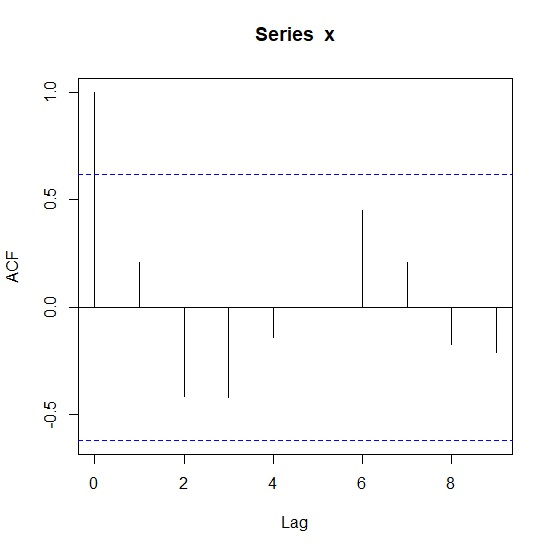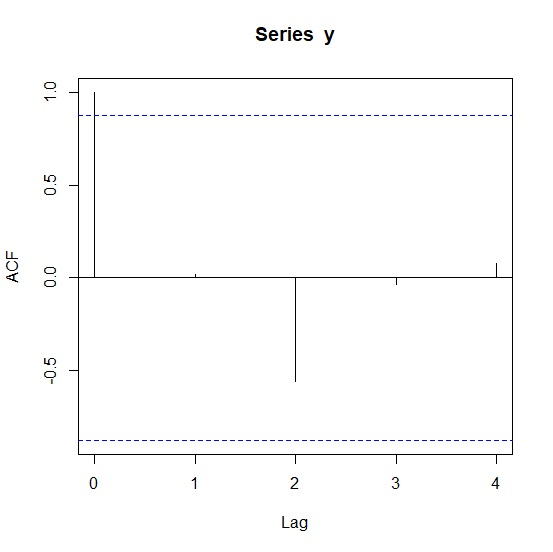# How to find the autocorrelation values from ACF plot in R?

The autocorrelation plot or ACF plot is a display of serial correlation in data that changes over time. The ACF plot can be easily created by using acf function.

For example, if we have a vector called V then we can create its autocorrelation plot by using the command acf(V). If we want to extract autocorrelation values then we would need to save the plot values in an object by using the below command. This will not create the plot.

Autocorrelation_x<-acf(x,plot=FALSE)

## Example 1

Consider the following snippet −

x<-rpois(10,2)
acf(x)

## Output

If you execute the above given snippet, it generates the following Output −To find the autocorrelation values from ACF plot in R, add the following code to the above snippet −

## Example

Autocorrelation_x<-acf(x,plot=FALSE)
Autocorrelation_x

## Output

If you execute all the above given snippets as a single program, it generates the following Output −

Autocorrelations of series ‘x’, by lag

0    1      2      3      4      5     6    7      8      9
1.000 0.207 -0.416 -0.420 -0.143 -0.002 0.451 0.209 -0.176 -0.211

## Example 2

Consider the following snippet −

y<-rpois(5,10)
acf(y)

## Output

If you execute the above given snippet, it generates the following Output −To find the autocorrelation values from ACF plot in R, add the following code to the above snippet −

## Example

Autocorrelation_y<-acf(y,plot=FALSE)
Autocorrelation_y

## Output

If you execute all the above given snippets as a single program, it generates the following Output −

Autocorrelations of series ‘y’, by lag

0    1     2     3      4
1.000 0.019 -0.557 -0.038 0.077